## 5.2 Dictionary

Important: We consider a coordinate system with X axis being positive on the left of the origin and Y axis being positive to the bottom of the origin, like for a screen with top-left pixel with (0,0) coordinate. This is important because sometimes I use term such as "left", "top" or "clockwise". If you intend to use another coordinate system, make sure to take care of that.

A path is described by a serie of knots joined by various type of interpolations. The library support 4 types of interpolation:

• straight-line,

• arc (SVG style),

• bezier curves of any degrees,

• catmull-rom.

See the constructor for each type of interpolation for more details. Each interpolations are implemented by a class and a set of CLOS methods. This way, it is easy to implement new interpolation scheme inside the library.

A path can be of 3 types:

• :open-polyline

• :closed-polyline

• :polygon (a implicitly closed and filled area.)

Paths of types :closed-polyline and :polygon are always closed implicitly by a straight line (or a custom interpolation) from the last knot to the first knot.

### 5.2.1 Points

A point is called a knot when it specify a position along the path (vs points used for Bezier control points.)

Function CREATE-POINT

• create-point x y => point

Function POINT-X

• point-x point => x

Function POINT-Y

• point-y point => y

Function P+

Syntax

• p+ point-1 point-2 => point-result

Arguments and Values:

• point-1 -- a point

point-2 -- a point

point-result -- a point

Description:

• Compute the translation of point-1 by point-2.

Function P-

• p- point-1 point-2 => point-result

Arguments and Values:

• point-1 -- a point

point-2 -- a point

point-result -- a point

Description:

• Compute the translation of point-1 by the opposite of point-2.

Notes:

• This is equivalent to (p+ point-1 (p* point-2 -1)).

Function P*

Syntax:

• p* point scale &optional (scale-y scale) => point-result

Arguments and Values:

• point -- a point

scale -- a real number

scale-y -- a real number

point-result -- a point

Description:

• Scale point by scale along the X axis, and scale-y along the Y axis. If scale-y is unspecified, then the point is scaled by the same amount in both axis.

Function POINT-ROTATE

Syntax:

• point-rotate point angle => point-result

Arguments and Values:

• point -- a point

angle -- an angle is radian

point-result -- a point

Description:

• Rotate point around origin by angle radian.

Function POINT-ANGLE

Syntax:

• point-angle point => angle

Arguments and Values:

• point -- a point

angle -- an angle in radian

Description:

• Compute the angle between the ray from the origin along the X axis and the ray from origin by the given point.

Notes:

• The angle verify the following form:

(point-rotate (make-point (point-norm point) 0) angle) => point

(If we do not consider floating point rounding errors.)

Function POINT-NORM

Syntax:

• point-norm point => distance

Arguments and Values:

• point -- a point

distance -- a real number

Description:

• Compute the distance of point from the origin.

Function POINT-DISTANCE

Syntax:

• point-distance point-1 point-2 => distance

Arguments and Values:

• point-1 -- a point

point-2 -- a point

distance -- a real number

Description:

• Compute the distance from point-1 to point-2.

Notes:

• This is equivalent to (point-norm (p- point-2 point-1)).

### 5.2.2 Paths

A path is made of knots and interpolations. All the points used to represent knots are immutable, they are never updated in place. (They're never destructively modified.)

Function CREATE-PATH

Syntax

• create-path type => path

Arguments and Values:

• type -- a symbol, among :open-polyline, :closed-polyline or :polygon.

Description:

• Create a new empty path of the specified type. To describe a path, the first knot is usually specified with path-reset, then the rest is specified by a serie of call to path-extend.

Function PATH-CLEAR

Syntax:

• path-clear path

Arguments and Values:

• path -- a path

Description:

• Remove all the knots from the path.

Function PATH-RESET

Syntax:

• path-reset path knot

Arguments and Values:

• path -- a path

knot -- a point

Description:

• Reset the path such that it starts at position given by knot. All other informations (except the type) are discarded. The implicit interpolation between the last knot and the first knot will be a straight line (which matter only if the type of the path is not :open-polyline).

Function PATH-EXTEND

Syntax:

• path-extend path interpolation knot

Arguments and Values:

• path -- a path

interpolation -- an instance of a class derived from interpolation-base

knot -- a point

Description:

• Extend the path from the last recorded knot to join the specified knot. The interpolation argument specify how both knots are joined.

If the path is still empty, then the interpolation argument specify the implicit interpolation between the last knot and the first knot if the path is of type :closed-polyline or :polygon.

Examples:

```(let ((path (create-path :polygon)))
(path-extend path (make-straight-line)
(make-point 10.0 10.0))
(path-extend path (make-straight-line)
(make-point 50.0 20.0))
(path-extend path (make-bezier-curve (list (make-point 80.0 30.0)))
(make-point 40.0 90.0))
(path-extend path (make-arc 90.0 90.0 :sweep-flag t)
(make-point 20.0 30.0))
(do-something path))
```

Function PATH-CONCATENATE

Syntax:

• path-concatenate path interpolation other-path

Arguments and Values:

• path -- a path

interpolation -- NIL or an interpolation

other-path -- a path

Description:

• Concatenate other-path to path. The other-path is kept unchanged.

If interpolation is not null, it will be used as the interpolation to join both path. Otherwise, the first interpolation of other-path is used.

Function PATH-REPLACE

Syntax:

• path-replace path other-path

Arguments and Values:

• path -- a path

other-path -- a path

Description:

• Replace path such that it is identical to other-path. The other-path is kept unchanged.

Function PATH-TYPE

Syntax:

• path-type path => type

Arguments and Values:

• path -- a path

type -- Either :open-polyline, :closed-polyline or :polygon.

Description:

• Give the type of path.

Function PATH-SIZE

Syntax:

• path-size path => size

Arguments and Values:

• path -- a path

size -- a non-negative integer

Description:

• Give the number of knots of path.

Function PATH-LAST-KNOT

Syntax:

• path-last-knot path => point

Arguments and Values:

• path -- a path

point -- NIL or a point

Description:

• Give the last knot in path. If the path is empty, NIL is returned.

Function PATH-CLONE

Syntax:

• path-clone path => new-path

Arguments and Values:

• path -- a path

new-path -- a path

Description:

• Return a path which is identical to path.

Function PATH-REVERSE

Syntax:

• path-reverse path

Arguments and Values:

• path -- a path

Description:

• Reverse the path in-place. See PATH-REVERSED to create a new path instead.

Function PATH-TRANSLATE

Syntax:

• path-translate path vector

Arguments and Values:

• path -- a path

vector -- a point

Description:

• Translate in place the path by the given vector.

Function PATH-ROTATE

Syntax:

• path-rotate path angle &optional center

Arguments and Values:

• path -- a path

angle -- an angle in radian

center -- NIL or a point

Description:

• Rotate in place the path, either around origin (if center is NIL) or around center, by angle radian.

Examples:

•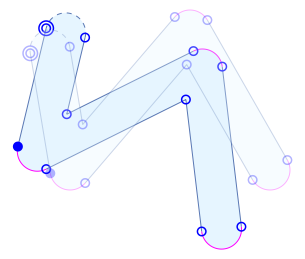```(let* ((paths (stroke-path (make-simple-path '((50 . 50) (70 . 170) (190 . 30) (270 . 170)))
40.0 :caps :round :inner-joint :miter :joint :round))
(paths-orig (mapcar #'path-clone paths)))
(dolist (path paths)
(path-rotate path 0.4 (make-point 100 80)))
(show-annotated-path paths :reference paths-orig))
```

Function PATH-SCALE

Syntax:

• path-scale path scale-x scale-y &optional center

Arguments and Values:

• path -- a path

scale-x -- a real number

scale-y -- a real number

center -- NIL or a point

Description:

• Scale in place the path by scale-x along the X axis and by scale-y along the Y axis. If center is not null, the path is scaled relatively to center.

### 5.2.3 Interpolations

Interpolations describe how knots are connected along a path.

An interpolation only specify the information needed in addition to the knots already on the path. For example, for a bezier curve of degree 2, only a single control point is necessary since the library will use the two knots around the interpolation to render it.

The 4 types of interpolations currently supported are represented in the picture below. The picture show a surface (in light cyan) described by 5 knots (blue circles.) In clockwise order, starting from the upper left knot (with double circles, which represent the first knot of a path, while the filled circle represent the second knot), we have the following interpolations:

• a straight line (in blue),

• a Bezier curve (in red) with a total of 5 control points (blue + red circles) meaning that it is a 4th degree curve,

• an arc (in purple) of a rotated ellipse,

• a Catmull-Rom spline (in green) with a total of 8 control points (blue + grey circles, outside and on the path).

The path is closed with an implicit straight line (dashed blue line.)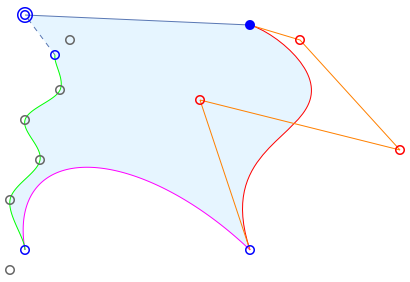The path was constructed with the following code:

```(let ((path (create-path :polygon)))
(path-reset path (make-point 25 15))
(path-extend path (make-straight-line) (make-point 250 25))
(path-extend path (make-bezier-curve (list (make-point 300 40)
(make-point 400 150)
(make-point 200 100)))
(make-point 250 250))
(path-extend path (make-arc 100 200 :x-axis-rotation -0.8)
(make-point 25 250))
(path-extend path (make-catmull-rom (make-point 10 270)
(list (make-point 10 200)
(make-point 40 160)
(make-point 25 120)
(make-point 60 90))
(make-point 70 40))
(make-point 55 55))
(show-annotated-path path))
```

Note: The show-annotated-path function is included in the library, but rely on an external program (image viewer.)

Function MAKE-STRAIGHT-LINE

Syntax

• make-straight-line => interpolation

Arguments and Values:

• None.

Description:

• Describe a straight line between the knots.

Function MAKE-ARC

Syntax:

• make-arc rx ry &key (x-axis-rotation 0.0) (large-arc-flag nil) (sweep-flag nil) => interpolation

Arguments and Values:

rx -- a number

ry -- a number

x-axis-rotation -- a number (angle in radian)

large-arc-flag -- boolean

sweep-flag -- boolean

Description:

Examples:

•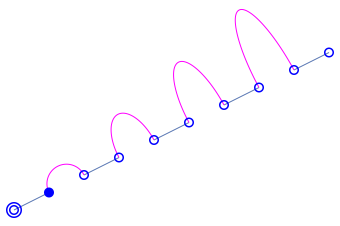```(let ((path (create-path :open-polyline)))
(path-reset path (make-point 20 300))
(path-extend path (make-straight-line) (make-point 70 275))
(path-extend path (make-arc 25 25 :x-axis-rotation -0.5 :sweep-flag t)
(make-point 120 250))
(path-extend path (make-straight-line) (make-point 170 225))
(path-extend path (make-arc 25 50 :x-axis-rotation -0.5 :sweep-flag t)
(make-point 220 200))
(path-extend path (make-straight-line) (make-point 270 175))
(path-extend path (make-arc 25 75 :x-axis-rotation -0.5 :sweep-flag t)
(make-point 320 150))
(path-extend path (make-straight-line) (make-point 370 125))
(path-extend path (make-arc 25 100 :x-axis-rotation -0.5 :sweep-flag t)
(make-point 420 100))
(path-extend path (make-straight-line) (make-point 470 75))
(show-annotated-path path))
```

(This example is inspired from the SVG documentation from W3.)

Function MAKE-CATMULL-ROM

Syntax:

• make-catmull-rom head control-points queue => interpolation

Arguments and Values:

head -- a point

control-points -- a list of points

queue -- a point

Description:

• This describe a Catmull-Rom interpolation. Such interpolations are normally described by a single list of points, but since two of them are already described by knots on the path, only the remaining points must be passed to the constructor. The effective list of points as processed by this interpolation method is:

(head knot1 control-points... knot2 queue)

where knot1 and knot2 are the knots on the path.

A short explanation of this type of splines is available here.

Examples:

•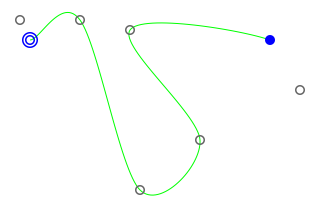```(let ((path (create-path :open-polyline)))
(path-reset path (make-point 30 40))
(path-extend path (make-catmull-rom (make-point 20 20)
(list (make-point 80 20)
(make-point 140 190)
(make-point 200 140)
(make-point 130 30))
(make-point 300 90))
(make-point 270 40))
(show-annotated-path path))
```

Function MAKE-BEZIER-CURVE

Syntax:

• make-bezier-curve control-points => interpolation

Arguments and Values:

• control-points -- a list of points

Description:

• This describe a Bezier curve interpolation. The degree of the curve depends on the number of control points passed as arguments. Since the knots around the interpolation are part of the Bezier curve, the degree of the curve is the length of the controls points list minus one.

Examples:

•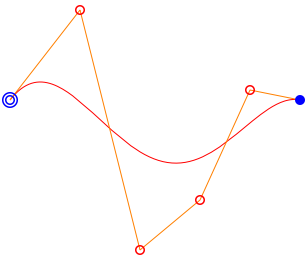```(let ((path (create-path :open-polyline)))
(path-reset path (make-point 10 100))
(path-extend path (make-bezier-curve (list (make-point 80 10)
(make-point 140 250)
(make-point 200 200)
(make-point 250 90)))
(make-point 300 100))
(show-annotated-path path))
```

Method INTERPOLATION-SEGMENT

Syntax:

• interpolation-segment interpolation k1 k2 function

Arguments and Values:

• interpolation -- an interpolation object

k1 -- a point

k2 -- a point

function -- a function taking a point as argument

Description:

• This method is used to produce a list of points along the interpolation. The k1, interpolation and k2 fully provides the necessary information for the interpolation to join k1 to k2. It calls FUNCTION for each computed points (but not for k1 and k2 themselves.)

Limitations:

• There are currently no ways to specify level of accuracy needed when performing theses computations, but this is planned (probably as a set of special variables.)

Method INTERPOLATION-CLONE

Syntax:

• interpolation-clone interpolation

Arguments and Values:

• interpolation -- an interpolation object

Description:

• Create a new interpolation object with the same information as the original.

Method INTERPOLATION-REVERSE

Syntax:

• interpolation-reverse interpolation

Arguments and Values:

• interpolation -- an interpolation object

Description:

• Change the interpolation such that it is reversed. It is used when reversing a whole path. In such case, each interpolations are reversed in the process, and this method must take care of it.

### 5.2.4 Iterators

A path iterator basically provide a way to iterate over all the knots and interpolations of a path. But this is also used to iterate over a "virtual" path (a path constructed as needed at each iteration.)

When the end of the path is reached, the iterator loop at the beginning. A marker is available to detect end of path.

When an iterator takes another iterator (instead of a path), it will be called a filter in this documentation.

Two functions are part of the iterator protocol:

Method PATH-ITERATOR-RESET

Syntax:

• path-iterator-reset iterator

Arguments and Values:

• iterator -- a path iterator

Description:

• Reset the iterator such that its state is like when it was first created.

Method PATH-ITERATOR-NEXT

Syntax:

• path-iterator-next iterator => interpolation knot end-p

Arguments and Values:

• iterator -- a path iterator

interpolation -- an interpolation

knot -- a point

end-p -- a boolean value

Description:

• Move the iterator to the next point, and returns information where the iterator is located. The interpolation is the one between the previous knot and the returned knot. If the knot was the last on the path, then end-p is true.

If the path is empty, then end-p is true and both interpolation and knot are NIL.

There is three iterators defined in the library:

Function PATH-ITERATOR

Syntax:

• path-iterator path => iterator

Arguments and Values:

• path -- a path

iterator -- a path iterator

Description:

• Create a new iterator over the given path.

Function PATH-ITERATOR-SEGMENTED

Syntax:

• path-iterator-segmented path &optional predicate => iterator

Arguments and Values:

• path -- a path

predicate -- a function

iterator -- a path iterator

Description:

• Create a new iterator, which automatically segment (convert interpolation to discrete path) for any interpolations which is not matched by the predicate.

The predicate must take one argument, which is the interpolation, and return a boolean value, which is true if the interpolation must be converted to a serie of straight line.

This iterator is used at various place internally to process a path, transforming interpolations not handled by some transformations.

Function FILTER-DISTINCT

Syntax:

• filter-distinct path &optional preserve-cyclic-end-p => iterator

Arguments and Values:

• path -- a path

preserve-cyclic-end-p -- a boolean value

iterator -- a path iterator

Description:

• Create a new iterator which discard any knot which is not distinct from the previous one.

Since iterators are cyclics, a problem may arise if both ends of the path are not distinct. If preserve-cyclic-end-p is true, then each ends will be returned by the iterator. Otherwise, only the first of the these knots will be returned and the last knot will be the knot before the last which is distinct.

### 5.2.5 Basic shape

Function to generate basic path shape are provided.

Function MAKE-CIRCLE-PATH

Syntax:

• make-circle-path cx cy radius &optional (radius-y radius) (x-axis-rotation 0.0) => path

Arguments and Values:

• cx -- a real number

cy -- a real number

radius -- a real number

radius-y -- a real number

x-axis-rotation -- a real number

Description:

• Create a new path describing a circle (or ellipse) centered at (cx,cy). Since SVG arc cannot describe an entire circle, such a path is made of 2 half-circles.

Examples:

•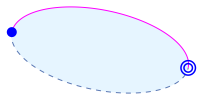```(let ((path (make-circle-path 100 50 90 40 0.2)))
(show-annotated-path path))
```

Function MAKE-RECTANGLE-PATH

Syntax:

• make-rectangle-path x1 y1 x2 y2 &key round round-x round-y => path

Arguments and Values:

• x1 -- a real number

y1 -- a real number

x2 -- a real number

y2 -- a real number

round -- NIL or a real number

round-x -- NIL or a real number

round-y -- NIL or a real number

Description:

• Create a new path describing a rectangle between (x1,y1) and (x2,y2), whose sides are parallel to axis. If round is specified, then each corner are rounded to the radius specified by this argument. The argument round-x and round-y allows to specify different radius for each axis.

Examples:

•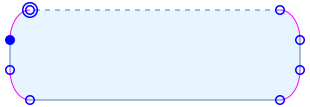```(let ((path (make-rectangle-path 10 10 300 100 :round-x 20 :round-y 30)))
(show-annotated-path path))
```

### 5.2.6 Transformations

Some transformations can produce more than one path. So, it was decided that by default most transformation return a list of path (possibly none, or usually only one.) And they can take either a single path or a list of path as parameter.

Function MAKE-DISCRETE-PATH

Syntax:

• make-discrete-path path => new-path

Arguments and Values:

• path -- a path

new-path -- the resulting path

Description:

• Produce a new path only composed of straight lines, effectively transforming all interpolations to a serie of segments.

Limitations:

• Several special variables can be modified to change the precision of the segmentation. Bezier curves are adaptively refined until meeting certain criterions, such as flatness or small angular step. Catmull-Rom splines are currently not rendered with adaptive method, but this is planned.

The special variables which affect the process are:

• *bezier-distance-tolerance*

Default is 0.5. For Bezier curves, this variable give the maximum distance allowed between the middle point of the curve and the straight line from both ends of the curve, at each refinement.

• *bezier-angle-tolerance*

Default is 0.05 radian. For Bezier curves, this variable give the maximum angle in radian allowed between the line from the first point and the middle point and the line from the last point and the middle point, at each refinement.

• *arc-length-tolerance*

Default is 1.0. Not used yet. This variable specify the maximum allowed length of segment used to describe an arc.

Examples:

• Consider the following stroked path (the original path is represented with low opacity on top of the resulting path) with a width of 100.0 unit:

```(let* ((path (make-simple-path '((80 . 80) (100 . 200) (250 . 80) (300 . 200))))
(stroked (stroke-path path 100.0 :joint :round
:inner-joint :miter
:caps :round)))
(show-annotated-path stroked :reference path))
```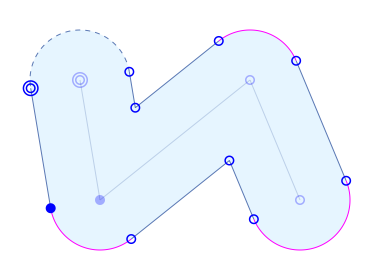It is described by straight lines and arcs.

Then when we transform it using make-discrete-path, we obtain the following path:

```(let* ((path (make-simple-path '((80 . 80) (100 . 200) (250 . 80) (300 . 200))))
(stroked (make-discrete-path (stroke-path path 100.0 :joint :round
:inner-joint :miter
:caps :round))))
(show-annotated-path stroked :reference path))
```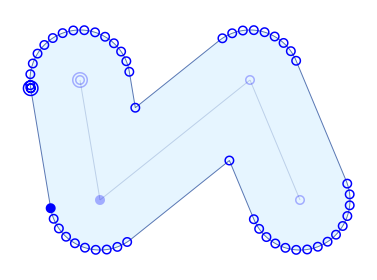Only straight lines remain. (Note that the path annotation had decimated many circles to represent dot, to get a better visual result, because there are many knots used to represent arc in reality.)

Function STROKE-PATH

Syntax:

• stroke-path paths thickness &key (caps :butt) (joint :none) (inner-joint :none) assume-type => new-paths

Arguments and Values:

• paths -- a path or a list of paths

thickness -- a number

caps -- one of :butt, :square or :round

joint -- one of :none, :miter or :round

inner-joint -- one of :none, :miter or :round

assume-type -- NIL or a path type.

Description:

• Returns a stroked path. The resulting paths will depend on the type of the input path. If it is a polygon, the result is the contour of it (assuming the polygon was described clockwise.) The assume-type argument allows to override the type of the path while deciding how to stroke it.

Examples:

• With :assume-type to :open-polyline: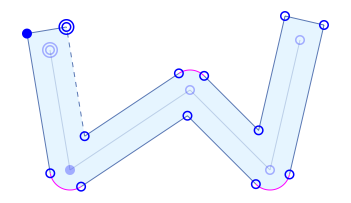With :assume-type to :closed-polyline: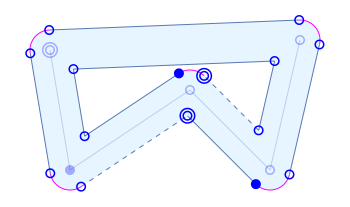With :assume-type to :polygon: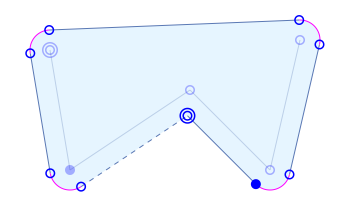Function DASH-PATH

Syntax:

• dash-path paths sizes &optional toggle-p (cycle-index 0) => new-paths

Arguments and Values:

• path -- a path or a list of paths

sizes -- an array of real number

toggle-p -- a boolean value

cycle-index -- a non-negative integer, smaller than the length of the sizes array.

Description:

• Produce a dashed path. FIXME.

If toggle-p is true, then the dashes are reversed (holes become dashes and vice versa.)

The value cycle-index specify where to loop in the sizes array once the end is reached.

Examples:

• ```(let ((path (create-path :open-polyline)))
(path-reset path (make-point 30 30))
(path-extend path (make-straight-line) (make-point 180 80))
(path-extend path (make-arc 80 80 :large-arc-flag t :sweep-flag t) (make-point 150 150))
(path-extend path (make-straight-line) (make-point 90 200))
(show-annotated-path (dash-path path #(80 50))
:reference path))
```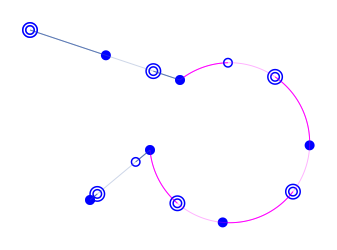Function ROUND-PATH

Syntax:

• round-path path max-radius => new-paths

Arguments and Values:

• path -- a path

max-radius -- a real number

Description:

• Not included in the current release yet. Round each part of a path up to the given radius. This is done only between consecutive straight line, while the rest of the path is kept inchanged. Note that it is not related to Bezier curves. The resulting path is composed only of straight line and arcs.

Examples:

• With max-radius set to 5.0: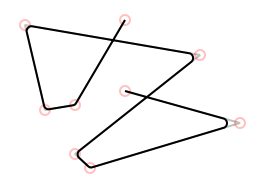With max-radius set to 10.0: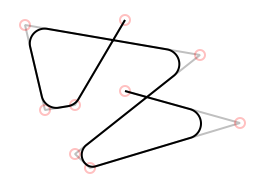With max-radius set to 1000.0: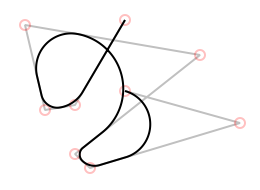Function CLIP-PATH

Syntax:

• clip-path paths x y dx dy => new-paths

Arguments and Values:

• paths -- a path or a list of paths

x -- a real number

y -- a real number

dx -- a real number

dy -- a real number

Description:

• Clip the path against the line by (x,y) with delta (dx,dy). Anything on the "right" of the line is split/discarded. (FIXME: right/left notion is vague.)

Function CLIP-PATH/PATH

Syntax:

• clip-path/path paths limit => new-path

Arguments and Values:

• paths -- a path or a list of paths

limit -- a convex path

Description:

• Clip the path against the convex polygon described by limit. If the limit path is not convex, then the clipping algorithm will not work properly (usually, by discarding more parts of the path than necessary.) Note also that limit path is first segmented, and that the algorithm take each segment one by one to clip the input path (which may be slow if the clipping path was made of complex interpolation.)

Examples:

• In this example, an open polyline is clipped against a rotated rectangle.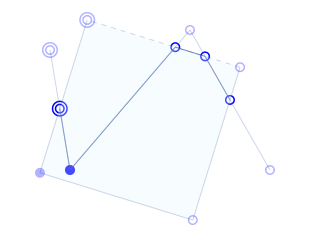```(let ((path (make-simple-path '((50 . 50) (70 . 170) (190 . 30) (270 . 170))))
(clipping (make-rectangle-path/center 140 120 80 80)))
(path-rotate clipping 0.3 (make-point 140 120))
(show-annotated-path (clip-path/path path clipping)
:reference (list path clipping)))
```
Generated by CL-Crock on 2010-09-25T15:20:58Z